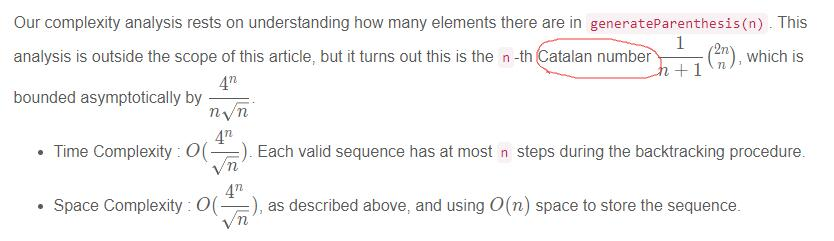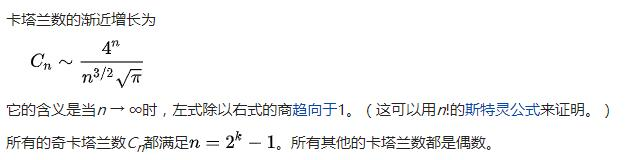## 1. 题目描述（中等难度）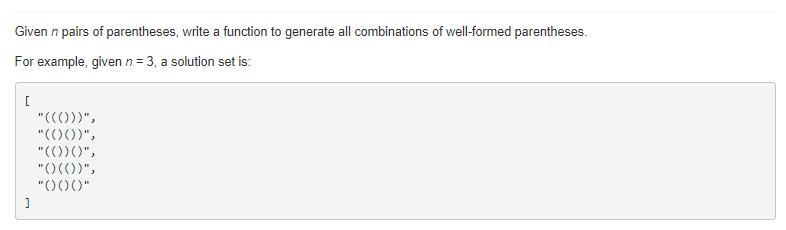# 解法一 暴力破解

public List<String> generateParenthesis(int n) {
List<String> combinations = new ArrayList();
generateAll(new char[2 * n], 0, combinations);
return combinations;
}

public void generateAll(char[] current, int pos, List<String> result) {
if (pos == current.length) {
if (valid(current))
} else {
current[pos] = '(';
generateAll(current, pos+1, result);
current[pos] = ')';
generateAll(current, pos+1, result);
}
}

public boolean valid(char[] current) {
int balance = 0;
for (char c: current) {
if (c == '(') balance++;
else balance--;
if (balance < 0) return false;
}
return (balance == 0);
}


# 解法二

public List<String> generateParenthesis(int n) {
List<String> ans = new ArrayList();
backtrack(ans, "", 0, 0, n);
return ans;
}

public void backtrack(List<String> ans, String cur, int left, int right, int n){
if (cur.length() == n * 2) {
return;
}
//左括号不要超过 n
if (left < n)
backtrack(ans, cur+"(", left+1, right, n);
//右括号不要超过左括号
if (right < left)
backtrack(ans, cur+")", left, right+1, n);
}


# 解法三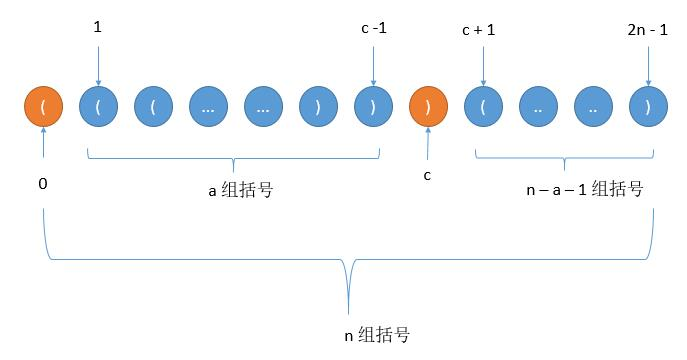a = 0，b = 2 对应 （）（（））以及 （）（）（） 两种情况，此时 c = 1。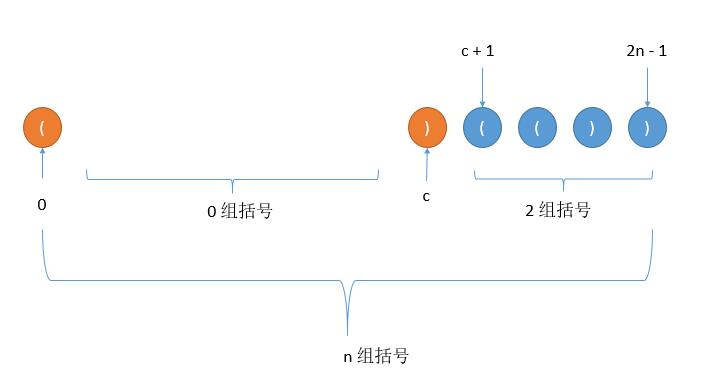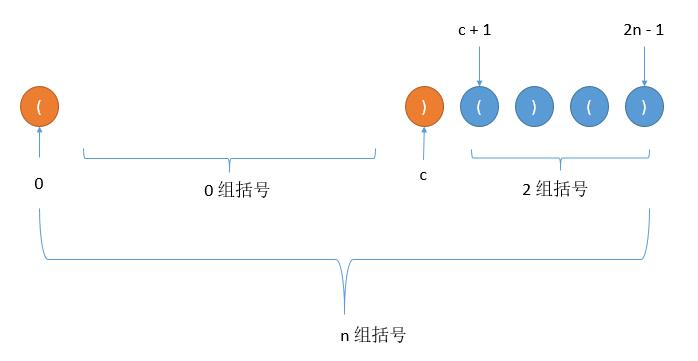a = 1，b = 1，对应 （（））（（）） 一种情况，此时 c = 3。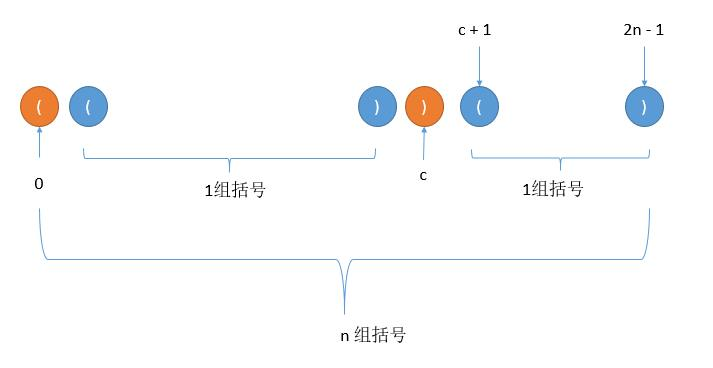a = 2，b = 0 对应 （（（）））， （（）（）） 两种情况，此时 c = 5。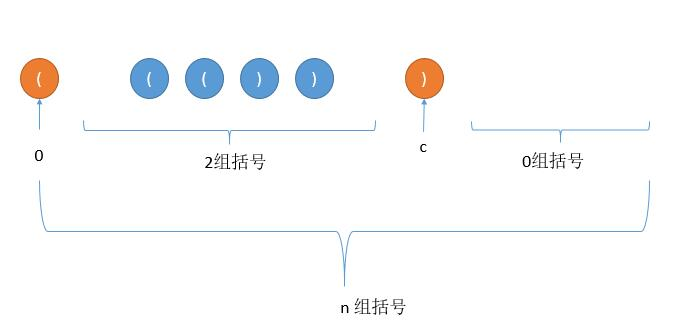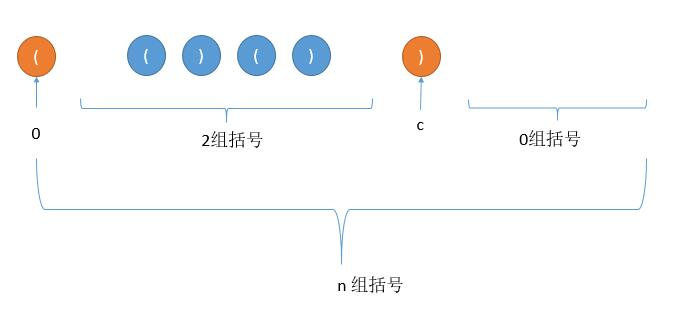public List<String> generateParenthesis(int n) {
List<String> ans = new ArrayList();
if (n == 0) {
} else {
for (int a = 0; a < n; a++)
for (String left: generateParenthesis(a))
for (String right: generateParenthesis(n-1-a))
ans.add("(" + left + ")" + right);
}
return ans;
}


# 扩展 卡塔兰数

ps：下边的 和 都是指两个数的和，不是你和我中的和。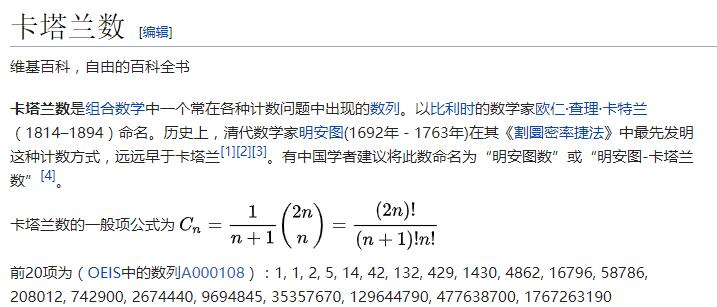2n 个人排队买票，其中 n 个人持 50 元，n 个人持 100 元。每张票 50 元，且一人只买一张票。初始时售票处没有零钱找零。请问这 2n 个人一共有多少种排队顺序，不至于使售票处找不开钱？

P = a1 a2 a3 ... an，其中 ai 是矩阵。根据乘法结合律，不改变矩阵的相互顺序，只用括号表示成对的乘积，试问一共有几种括号化方案？

n 个结点可构造多少个不同的二叉树？

... ...